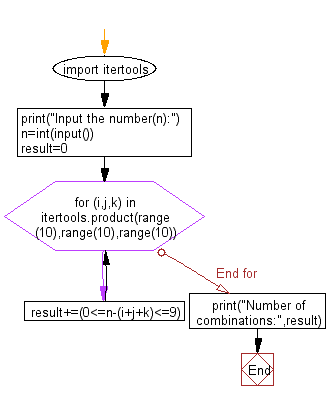﻿ Python: Find the number of combinations of a,b,c and d - w3resource# Python: Find the number of combinations of a,b,c and d

## Python Basic - 1: Exercise-37 with Solution

Write a Python program which reads an integer n and find the number of combinations of a,b,c and d (0 ≤ a,b,c,d ≤ 9) where (a + b + c + d) will be equal to n.

Input:
n (1 ≤ n ≤ 50)

Sample Solution:

Python Code:

``````import itertools
print("Input the number(n):")
n=int(input())
result=0
for (i,j,k) in itertools.product(range(10),range(10),range(10)):
result+=(0<=n-(i+j+k)<=9)
print("Number of combinations:",result)
``````

Sample Output:

```Input the number(n):
15
Number of combinations: 592
```

Flowchart:Python Code Editor:

Have another way to solve this solution? Contribute your code (and comments) through Disqus.

What is the difficulty level of this exercise?

Test your Python skills with w3resource's quiz

﻿

## Python: Tips of the Day

Checks if the given number falls within the given range.

Example:

```def tips_range(n, start, end = 0):
return start <= n <= end if end >= start else end <= n <= start
print(tips_range(2, 4, 6))
print(tips_range(4, 8))
print(tips_range(1, 3, 5))
print(tips_range(1, 3))
```

Output:

```False
True
False
True
```